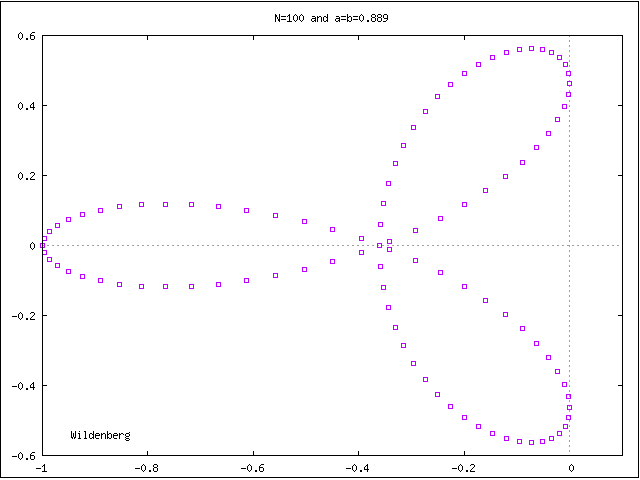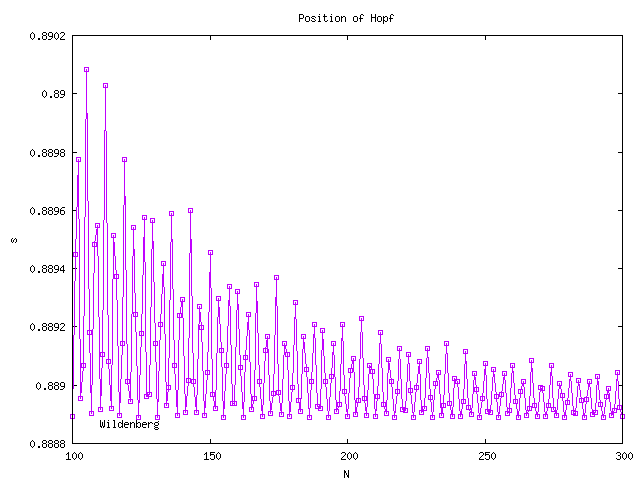# J. C. Wildenberg, J. C. Sprott, and J. A. Vano

Departments of Physics and Mathematics, University of Wisconsin, Madison, WI 53706, USA
November 9, 2004 (modified February 26, 2005)

Chaos has been observed in the spatiotemporal Lotka-Volterra model described by

dxi/dt = xi(1 - axi-2 - xi - bxi+1)

for a = b = 1 and i = 1 to N with N > 65 and periodic boundary conditions. The system is described in detail in another publication. The system exhibits a quasiperiodic route to chaos with a first Hopf bifurcation that occurs at a value of approximately a = b = 0.889. Below that value, the orbit approaches the equilibrium at xi = 1/(a + b + 1) for all i. For N = 100 the eigenvalues form a trefoil at the Hopf bifurcation:If we set s = a = b then s is a parameter that multiplies the off-diagonal elements of the interaction matrix. This animation shows how the eigenvalues grow as s increases with N = 100. Using this parameter we can examine the routes to chaos by changing s or by changing N. For each value of N the Hopf bifurcation occurs at a different value of s. The exact position oscillates but is damped as N increases:The eigenvalues for a given s value fall on a curve that does not change with N. The number of eigenvalues, however, does change, and it is a rotation of these eigenvalues to accommodate the new ones that produces the change in the position of the Hopf bifurcation. As N increases the curve becomes filled, so that the oscillations shrink. In the limit of large N the s value at the Hopf bifurcation is asymptotic to ~0.8888916. This animation shows the rotation of the eigenvalues as new ones are added (emanating from the fixed point).